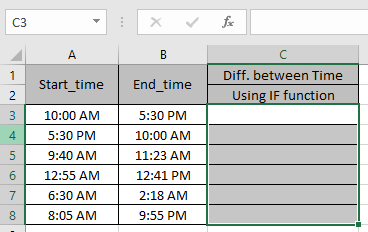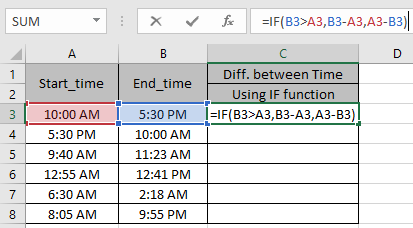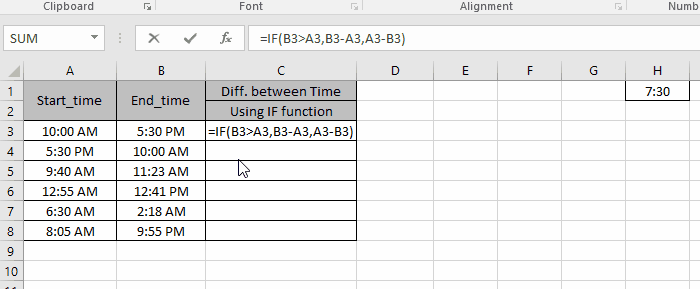# How to Calculate hours between time in Excel

In this article, we will learn how to get hours between two given time in Excel.

In simple words, we need to find the time elapsed between end_time & start_time
A simple formula to get the result

=End_time - Start_time

But there is a problem that if the excel takes the difference between the two in negative or more than 24 hours. So it's advised to use the date with time. Then this formula will get your results.

Here we have just start_time & end_time and we need to find the difference between the two considering the two times are of the same date. So first we will compare the two times using IF function. Let’s learn this through an example shown below.Use the IF formula

=IF( B3 > A3, B3 - A3, A3 - B3 )

Explanation:
The formula compares the two times. So as to keep the answers to positive as time elapsed between times can’t be negative it returns value_if_true or value_if_false.Copy the formula in other cells using Ctrl + D shortcut.As you can see the time elapsed between the two given times is in column C.

Hope you learn how to get the time between two times in Excel. Explore more articles here on Date & Time. Feel free to state your queries below. We will help you.

Related Articles:

How to Convert Date and Time from GMT to CST in Excel

How to Calculate Minutes Between Dates & Time In Microsoft Excel

How to take Time difference in hours as decimal value in Excel

How to convert decimal Seconds into time format in Excel

How to Convert Time to Decimal in Excel

Popular Articles:

50 Excel Shortcuts to Increase Your Productivity

How to use the VLOOKUP Function in Excel

How to use the COUNTIF function in Excel

How to use the SUMIF Function in Excel

Terms and Conditions of use

The applications/code on this site are distributed as is and without warranties or liability. In no event shall the owner of the copyrights, or the authors of the applications/code be liable for any loss of profit, any problems or any damage resulting from the use or evaluation of the applications/code.